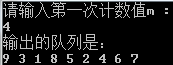# C语言解决约瑟夫环问题 详细注释

/*约瑟夫环*/
#include <stdio.h>
#include <stdlib.h>

#define N 9  //环中人的个数
#define OVERFLOW 0
int KeyW[N]={4,7,5,9,3,2,6,1,8};  //每个人手中的密码

typedef struct LNode{  //定义循环链表
int keyword;
struct LNode * next;

void Joseph(LinkList p,int m,int x){  //x为总人数，m为删除的那个人手中的密码，起始的m为第一个人手中的密码
int i;
if(x==0)return;
q=p;
m%=x;
if(m==0)m=x;
for(i=1;i<=m;++i){  //找到第m个人  p指向要删除的人的前一人 q指向要删除的人
p=q;
q=p->next;
}
p->next=q->next;  //删除q
i=q->keyword;  //得到下一个要删除的人的位置
printf("%d ",q->keyword);
free(q);
Joseph(p,i,x-1);  //递归调用 总人数减1，输入新的密码
}

int main()
{
int i,m;
for(i=1;i<9;++i){
q->keyword=KeyW[i];  //对q的keyword进行赋值
p->next=q;  //将q赋给p的next
p=q;  //p要指向尾部，将q赋给p
}